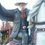# Divisors of 999...999 using division in a unusual way

Which integers can be multiplied by another integer so the product is in the form of 999....999?

To answer this question, will use some concepts, though I don't have a rigorous proof of these(I will update this note if I find something), a irreducible fraction:

1) Will only have terminating decimals if the denominator can be written as product of powers of two and five;

"Proof": $\frac{n}{2^a\times5^b}=\frac{n}{2^a\times5^b}\times \frac{10^c}{10^c}=\frac{n\times2^{c-a}\times5^{c-b}}{10^c}$, with $c=max(a,b)$

2) Will only have non-terminating decimals if the denominator is not a multiple of two and five, but is a multiple of another prime number;

3) Will have both terminating(non-repeating) and non-terminating decimals if the denominator is a multiple of 2 or 5, and is a multiple of another prime;

I will use the second case; It is possible to revert it back to a fraction:

$0.\overline{\text{(part that repeats)}}=\frac{\text{part that repeats}}{\text{a sequence of 9 with the same number of digits as the part that repeats}}$

So there is a way to transform a(irreducible) fraction whose denominator is not a multiple of two and five to a fraction whose denominator is a sequence of nines

$\frac{1}{n}=\frac{a}{99...999}$ if n is not a multiple of 2 nor 5(if $n=1$(not a multiple of another prime) then it is trivial)

$n=\frac{99...999}{a}$

$n\times a=99...999$

With this, I can, for instance, find the number that, multiplied by 17, will return a sequence of nines:

$\frac{1}{17}=0.\overline{0588235294117647}=\frac{588235294117647}{9999999999999999}$

$17=\frac{9999999999999999}{588235294117647}$

$17\times588235294117647=9999999999999999$Note by Matheus Jahnke
3 years, 8 months ago

This discussion board is a place to discuss our Daily Challenges and the math and science related to those challenges. Explanations are more than just a solution — they should explain the steps and thinking strategies that you used to obtain the solution. Comments should further the discussion of math and science.

When posting on Brilliant:

• Use the emojis to react to an explanation, whether you're congratulating a job well done , or just really confused .
• Ask specific questions about the challenge or the steps in somebody's explanation. Well-posed questions can add a lot to the discussion, but posting "I don't understand!" doesn't help anyone.
• Try to contribute something new to the discussion, whether it is an extension, generalization or other idea related to the challenge.

MarkdownAppears as
*italics* or _italics_ italics
**bold** or __bold__ bold
- bulleted- list
• bulleted
• list
1. numbered2. list
1. numbered
2. list
Note: you must add a full line of space before and after lists for them to show up correctly
paragraph 1paragraph 2

paragraph 1

paragraph 2

[example link](https://brilliant.org)example link
> This is a quote
This is a quote
    # I indented these lines
# 4 spaces, and now they show
# up as a code block.

print "hello world"
# I indented these lines
# 4 spaces, and now they show
# up as a code block.

print "hello world"
MathAppears as
Remember to wrap math in $$ ... $$ or $ ... $ to ensure proper formatting.
2 \times 3 $2 \times 3$
2^{34} $2^{34}$
a_{i-1} $a_{i-1}$
\frac{2}{3} $\frac{2}{3}$
\sqrt{2} $\sqrt{2}$
\sum_{i=1}^3 $\sum_{i=1}^3$
\sin \theta $\sin \theta$
\boxed{123} $\boxed{123}$

Sort by:

Every integers except those divisible by 2 and 5, can be multiplied in such a way.

For any integer $q$ ,

Consider order of $10 (mod q)$,

denote it $k$ .

Then $10^k \equiv 1 \Rightarrow 99999.....9 \equiv 0 (mod q)$

But we can generalise the result, to For any integer $q$,

Let $\alpha, \beta$ be the maximum power of 2,5 dividing $q$ respectively.

Then there always exists a number $999...9990000.....000$ with number of zero $= max (\alpha , \beta )$.

- 3 years, 7 months ago

Well, actually, you can generalize even more, when you consider another base system, here I used another way Euler Theorem to make this more rigorous: https://brilliant.org/discussions/thread/divisors-of-9999999-in-the-right-way/?ref_id=1339441

- 3 years, 4 months ago